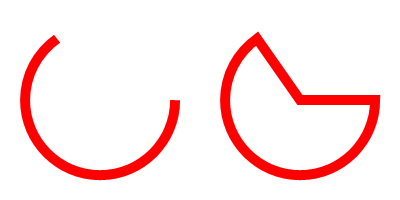Syntax

Description
Add a circle (or a partial circle) to the vector drawing path.

By default, this function ends the current figure in the path and adds the circle as an unconnected figure to the path (full circles are marked as closed). This behavior can be changed with the appropriate flags.

Parameters

 x.d, y.d Specifies the center point for the circle. Radius.d Specifies the radius for the circle. StartAngle.d, EndAngle.d (optional) Specifies the angle for start and end of the circle in degrees. The angle 0 marks at the positive X axis. The defaults are 0 and 360 degrees respectively. Flags (optional) This can be a combination of the following values: ``` #PB_Path_Default : No special behavior (default value) #PB_Path_Relative : The positions are relative to the last cursor position. #PB_Path_Connected : The circle is connected to the existing path with a line and not automatically a closed figure. #PB_Path_CounterClockwise : The drawing direction between the start/end angles is counter-clockwise. ```

Return value

None.

Remarks

This function is indended for drawing standaline circles or arcs. To draw figures with rounded corners, the AddPathArc() function can be used which automatically calculates the proper angles and center point to draw rounded corners.

Example

```  If OpenWindow(0, 0, 0, 400, 200, "VectorDrawing", #PB_Window_SystemMenu | #PB_Window_ScreenCentered)

If StartVectorDrawing(CanvasVectorOutput(0))

; partial circle

; partial circle with lines to the center
MovePathCursor(300, 100)
AddPathCircle(300, 100, 75, 0, 235, #PB_Path_Connected)
ClosePath()

VectorSourceColor(RGBA(255, 0, 0, 255))
StrokePath(10)

StopVectorDrawing()
EndIf
EndIf
```